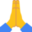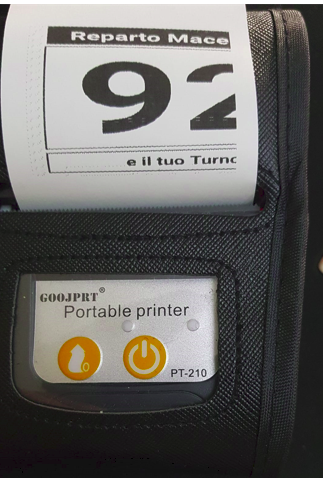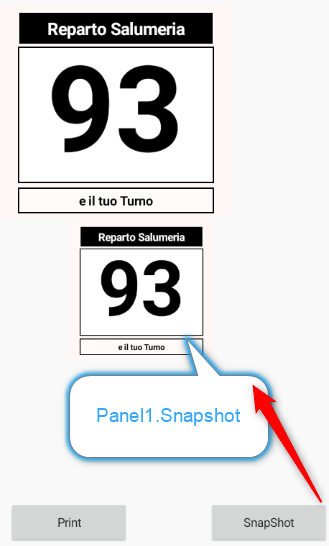# ItalianCreare Un Box o linea con Stampante bluetooth

#### Xfood

##### Expert
Buongiorno A tutti,
sto utilizzando questa classe,

e nonostante nel codice ci sono diverse funzioni per creare triangoli, box ecc, non riesco a creare un box, un cerchio oppure un tringolo
ho cercato e non ho trovato nessun esempio per realizzarerlo,
vorrei stampare una cosa del generequalcuno puo indicarmi con un esempio come stampare una cosa del genere?
Grazie mille a tutti

#### Star-Dust

##### Expert
Longtime User
Devi andare in grafica per una cornice. Poi la scritta in reverse, non so se c'è un comando altrimenti sempre in grafica.

Meglio se crei un immagine e poi la stampi in grafica. Certo rallenta il tutto.
Almeno io lo farei cosi, non so se ci sono caratteri speciali che puoi usare per realizzare una cornice cosi grossa.

•Solutions Development

#### Xfood

##### Expert
la scritta in reverse mi viene cosi:Longtime User

#### Xfood

##### Expert
Anziché lo spazio prova qualche altro carattere vuoto
buona idea, oggi provo ...

nella classe si trovano queste funzioni,
qualcuno ha provato ad usarle, se si come.....
B4X:
``````' This is a higher level method that builds the Int values to pass to CreateCustomCharacter in the shapes array
' Create the value to draw a line in a custom character
' The line starts at X0,Y0 and ends at X1,Y1
Public Sub CreateLine(x0 As Int, y0 As Int, x1 As Int, y1 As Int) As Int
Dim line As Int = 0
line = line + Bit.ShiftLeft(Bit.And(0xf,x0), 24)
line = line + Bit.ShiftLeft(Bit.And(0x1f,y0), 16)
line = line + Bit.ShiftLeft(Bit.And(0xf,x1), 8)
line = line + Bit.And(0x1f,y1)
Return line
End Sub

' This is a higher level method that builds the Int values to pass to CreateCustomCharacter in the shapes array
' Create the value to draw a circle in a custom character
' The circle is centred on X1,Y1 and the quadrants to draw are bit ORed together
' UpperRight = 0x1, LowerRight = 0x2, LowerLeft = 0x4, Upper Left = 0x8
Public Sub CreateCircle(radius As Int, quadrants As Int, x1 As Int, y1 As Int, fill As Boolean) As Int
Dim circle As Int = 0x20000000
If fill Then circle = circle + 0x80000000
circle = circle + Bit.ShiftLeft(radius, 24)
circle = circle + Bit.ShiftLeft(quadrants, 16)
circle = circle + Bit.ShiftLeft(x1, 8)
circle = circle + y1
Return circle
End Sub

' This is a higher level method that builds the Int values to pass to CreateCustomCharacter in the shapes array
' Create the value to draw a triangle in a custom character
' The triangles corners are at X0,Y0 X1,Y1 and X2,Y2
Public Sub CreateTriangle(x0 As Int, y0 As Int, x1 As Int, y1 As Int, x2 As Int, y2 As Int, fill As Boolean) As Int
Dim triangle As Int = 0x30000000
If fill Then triangle = triangle + 0x80000000
triangle = triangle + Bit.ShiftLeft(Bit.And(0xf,x0), 24)
triangle = triangle + Bit.ShiftLeft(Bit.And(0x1f,y0), 16)
triangle = triangle + Bit.ShiftLeft(Bit.And(0xf,x1), 8)
triangle = triangle + Bit.And(0x1f,y1)
triangle = triangle + Bit.ShiftLeft(Bit.And(0xf,x2), 12) ' extra X
triangle = triangle + Bit.ShiftLeft(Bit.And(0x7,y2), 5) ' extra Y lsbits * 3
triangle = triangle + Bit.ShiftLeft(Bit.And(0x18,y2), 18) ' extra Y msbits * 2
Return triangle
End Sub

' This is a higher level method that builds the Int values to pass to CreateCustomCharacter in the shapes array
' Create the value to draw a box in a custom character
' The box top left start is X0,Y0 and bottom right is X1,Y1
Public Sub CreateBox(x0 As Int, y0 As Int, x1 As Int, y1 As Int, fill As Boolean) As Int
Dim box As Int = 0x10000000
If fill Then box = box + 0x80000000
box = box + Bit.ShiftLeft(Bit.And(0xf,x0), 24)
box = box + Bit.ShiftLeft(Bit.And(0x1f,y0), 16)
box = box + Bit.ShiftLeft(Bit.And(0xf,x1), 8)
box = box + Bit.And(0x1f,y1)
Return box
End Sub

'-----------------------------------------
' Private custom character drawing methods
'-----------------------------------------

Private Sub PlotTriangle(x0 As Int, y0 As Int, x1 As Int, y1 As Int, x2 As Int, y2 As Int, points(,) As Byte, Fill As Int)
' This is a pretty crude algorithm, but it is simple, works and it isn't invoked often
PlotLine(x0, y0, x1, y1, points)
PlotLine(x1, y1, x2, y2, points)
PlotLine(x2, y2, x0, y0, points)
If Fill > 0 Then
FillTriangle(x0, y0, x1, y1, x2, y2, points)
End If
End Sub

Private Sub FillTriangle(x0 As Int, y0 As Int, x1 As Int, y1 As Int, x2 As Int, y2 As Int, points(,) As Byte)
' first sort the three vertices by y-coordinate ascending so v0 Is the topmost vertice */
Dim tx, ty As Int
If y0 > y1 Then
tx = x0 : ty = y0
x0 = x1 : y0 = y1
x1 = tx : y1 = ty
End If
If y0 > y2 Then
tx = x0 : ty = y0
x0 = x2 : y0 = y2
x2 = tx : y2 = ty
End If
If y1 > y2 Then
tx = x1 : ty = y1
x1 = x2 : y1 = y2
x2 = tx : y2 = ty
End If

Dim dx0, dx1, dx2 As Double
Dim x3, x4, y3, y4 As Double
Dim inc As Int

If y1 - y0 > 0 Then    dx0=(x1-x0)/(y1-y0) Else    dx0=0
If y2 - y0 > 0 Then dx1=(x2-x0)/(y2-y0) Else dx1=0
If y2 - y1 > 0 Then dx2=(x2-x1)/(y2-y1) Else dx2=0
x3 = x0 : x4 = x0
y3 = y0 : y4 = y0
If dx0 > dx1 Then
While
Do While y3 <= y1
If x3 > x4 Then inc = -1 Else inc = 1
For x = x3 To x4 Step inc
points(x, y3) = 1
Next
y3 = y3 + 1 : y4 = y4 + 1 : x3 = x3 + dx1 : x4 = x4 + dx0
Loop
x4=x1
y4=y1
Do While y3 <= y2
If x3 > x4 Then inc = -1 Else inc = 1
For x = x3 To x4 Step inc
points(x ,y3) = 1
Next
y3 = y3 + 1 : y4 = y4 + 1 : x3 = x3 + dx1 : x4 = x4 + dx2
Loop
Else
While
Do While y3 <= y1
If x3 > x4 Then inc = -1 Else inc = 1
For x = x3 To x4 Step inc
points(x, y3) = 1
Next
y3 = y3 + 1 : y4 = y4 + 1 : x3 = x3 + dx0 : x4 = x4 +dx1
Loop
x3=x1
y3=y1
Do While y3<=y2
If x3 > x4 Then inc = -1 Else inc = 1
For x = x3 To x4 Step inc
points(x, y3) = 1
Next
y3 = y3 + 1 : y4 = y4 + 1 : x3 = x3 + dx2 : x4 = x4 + dx1
Loop
End If
End Sub

Private Sub PlotBox(x0 As Int, y0 As Int, x1 As Int, y1 As Int, points(,) As Byte, Fill As Int)
' This is a pretty crude algorithm, but it is simple, works and itsn't invoked often
PlotLine(x0, y0, x0, y1, points)
PlotLine(x0, y0, x1, y0, points)
PlotLine(x1, y0, x1, y1, points)
PlotLine(x0, y1, x1, y1, points)
If Fill > 0 Then
For x = x0 To x1
PlotLine(x, y0, x, y1, points)
Next
End If
End Sub

Private Sub PlotCircle(radius As Int, quadrants As Int, x1 As Int, y1 As Int, points(,) As Byte, fill As Int)
' This is a pretty crude algorithm, but it is simple, works and itsn't invoked often
Dim mask As Int = 1
For q = 3 To 0 Step -1
For i = q*90 To q*90+90 Step 1
Dim x,y As Double
If fill > 0 Then
PlotLine(x1, y1, x, y, points)
Else
points(Round(x), Round(y)) = 1
End If
Next
End If
Next
End Sub

' Bresenham's line algorithm - see Wikipedia
Private Sub PlotLine(x0 As Int, y0 As Int, x1 As Int, y1 As Int, points(,) As Byte )
If Abs(y1 - y0) < Abs(x1 - x0) Then
If x0 > x1 Then
PlotLineLow(x1, y1, x0, y0, points)
Else
PlotLineLow(x0, y0, x1, y1, points)
End If
Else
If y0 > y1 Then
PlotLineHigh(x1, y1, x0, y0, points)
Else
PlotLineHigh(x0, y0, x1, y1, points)
End If
End If
End Sub

Private Sub PlotLineHigh(x0 As Int, y0 As Int, x1 As Int, y1 As Int, points(,) As Byte )
Dim dx As Int = x1 - x0
Dim dy  As Int = y1 - y0
Dim xi As Int = 1
If dx < 0 Then
xi = -1
dx = -dx
End If
Dim D As Int = 2*dx - dy
Dim x As Int = x0
For y = y0 To y1
points(x,y) = 1
If D > 0 Then
x = x + xi
D = D - 2*dy
End If
D = D + 2*dx
Next
End Sub

Private Sub    PlotLineLow(x0 As Int, y0 As Int, x1 As Int,y1 As Int, points(,) As Byte )
Dim dx As Int = x1 - x0
Dim dy As Int = y1 - y0
Dim yi As Int = 1
If dy < 0 Then
yi = -1
dy = -dy
End If
Dim D As Int = 2*dy - dx
Dim y As Int = y0
For x = x0 To x1
points(x,y) = 1
If D > 0 Then
y = y + yi
D = D - 2*dx
End If
D = D + 2*dy
Next
End Sub``````

#### Star-Dust

##### Expert
Longtime User
Per lo spazio sostituisci con _ e vedi se funziona

••Solutions Development and Xfood

#### Xfood

##### Expert
Per lo spazio sostituisci con _ e vedi se funziona
sembra che funziona, in realta viene stampanto il trattino basso _ ma sembra un effetto ottico che manca un pochino di
di neretto, in mancanza di altro va bene cosi.

•Star-Dust

#### Star-Dust

##### Expert
Longtime User
Per le barre laterali c'è un carattere ma non ricordo quale, prova a vedere la mappa dei caratteri di Windows

•Xfood

#### Lello1964

##### Well-Known Member
Longtime User
Buongiorno A tutti,
sto utilizzando questa classe,

e nonostante nel codice ci sono diverse funzioni per creare triangoli, box ecc, non riesco a creare un box, un cerchio oppure un tringolo
ho cercato e non ho trovato nessun esempio per realizzarerlo,
vorrei stampare una cosa del genere
View attachment 130392

qualcuno puo indicarmi con un esempio come stampare una cosa del genere?
Grazie mille a tutti
non stampa la è accentata oppure è un errore ?

#### Xfood

##### Expert
non stampa la è accentata oppure è un errore ?
se provi con questo set di caratte funziona
Printer1.WriteString2(Printer1.BOLD & "prova stampa è é ","CP819")

•Star-Dust

#### Star-Dust

##### Expert
Longtime User
se provi con questo set di caratte funziona
Printer1.WriteString2(Printer1.BOLD & "prova stampa è é ","CP819")
Dipende molto dalla stampante. In quelle cinesi spesso devi pensare anche per il simbolo euro. Quindi devi cambiare codifica, tabella eccc per ottenere certi caratteri

#### Xfood

##### Expert
Buongiorno,
sto impazzendo nel creare dei box da stampare ,
ho seguito il consiglio di @Star-Dust di creare una bitmap
ed eseguire uno Panel1.Snapshot, ma purtroppo stampa un questo modo,
non riesco a fare stampare la bitmap piu a sinistra, qualche idea?
tra laltro posso solo modificare il valore 300 che sarebbe ( credo ) la dimensione verticare
mentre il valore 576 se lo modifico l'immaggine viene stravolta .
allego progetto di esempio, qualcuno potrebbe provarlo e capire perche non riesco
a stampare correttamente la bitmap...
Grazie

B4X:
``````Dim bmp As Bitmap
bmp.InitializeResize(File.DirInternal, "Test22.png", 576,300, False) 'ignore 576``````lo snapshot funziona
ecco come viene#### Attachments

• Ticket.zip
25.4 KB · Views: 53

#### LucaMs

##### Expert
Longtime User
Purtroppo non posso aiutarti, @Xfood, ma ho voglia di farti qualche domanda (e un'osservazione: XFood e banco salumeria).

Ho visto quella stampatina sul Web e sono rimasto sorpreso del prezzo: si trova intorno ai 20€ (ma anche 50€) + spese di spedizioneQuanto costa la carta?

Ma la domanda principale è: stampi il numero assegnato a un cliente, con la scritta: "è il tuo turno" (a proposito, metti la "e" accentata, "è") ma non capisco la cosa, visto che "suppongo" che i clienti non abbiano quella stampante (a meno che non la fornisca il commerciante!).

P.S. Forse sono finalmente sveglio; suppongo che si intenda: "il 93 sarà il tuo turno", non che venga avvertito che sia il suo turno in quel momentoLast edited:

#### Xfood

##### Expert
Buongiorno @LucaMs
Xfood nasce tanto tempo fa, circa 32 anni fa
Quando non trovavo lavoro, e dicevo fra me e me, cosa devo fare per Mangiare tutti i giorni? Cosi iniziai a studiare informatica per Mangiare , e da li (xfood) oggi posso dire che ci sono riuscito, mangio tutti i giorni grazie all'informatica.

La stampante in questione l'ho presa per fare delle prove, comunque utilizza sempre il linguaggio ESC/POS, ottima per fare le prove, con un costo di 32 euro spedizioni comprese , ho preso la stampante da aliexpress compresa di custodia da cintura.
Il prezzo dei rotoli onestamente non lo so
( oggi chiedo ) utilizza i rotoli che si mettono nei normali pos ( pagamento elettronico) di tutti gli esercenti, qundi carta termica 58mm x 15Mt .

Per la e è hai ragione, inizialmente avevo il problema che non veniva riconosciuta dalla stampante, e poi con questa impostazione
Printer1.WriteString2(Printer1.BOLD & "prova stampa è é ","CP819") ho risolto, ma l'esempio e' rimasto ancora bacato.•LucaMs

#### Lello1964

##### Well-Known Member
Longtime User
manca sempre l'accento sulla e

#### Xfood

##### Expert
manca sempre l'accento sulla e
Direi che non e' il mio problema di oggi..#### LucaMs

##### Expert
Longtime User
Purtroppo non posso aiutarti, @Xfood, ma
... ed il motivo è che non ho una stampante di quel genere.
Sto, però, dando un'occhiata al tuo sorgente...

(mentre stavo scrivendo questo post, è arrivato il tuo, per cui...
Xfood nasce tanto tempo fa, circa 32 anni fa
Quando non trovavo lavoro, e dicevo fra me e me, cosa devo fare per Mangiare tutti i giorni? Cosi iniziai a studiare informatica per Mangiare , e da li (xfood) oggi posdo dire che ci sono riuscito, mangio tutti i giorni grazie all'informatica.
Allora sarebbe stato meglio iFoodEh, io oggi con l'informatica non mangio; fortunatamente, presto non avrò la necessità di mangiare•Xfood

#### LucaMs

##### Expert
Longtime User
Sto, però, dando un'occhiata al tuo sorgente...
Hai:
B4X:
``````    Dim m As Bitmap =  Panel1.Snapshot
m.Resize(200,100,True)``````
ma Panel1 è impostato come 270x250, nel Designer, e con AutoScaleAll, mentre il resizing lo fai su 200x100 (e senza specificare dip).
Inoltre, le View non toccano i bordi di Panel1, ecco perché...
non riesco a fare stampare la bitmap piu a sinistra, qualche idea?

#### Xfood

##### Expert
... ed il motivo è che non ho una stampante di quel genere.
Sto, però, dando un'occhiata al tuo sorgente...

(mentre stavo scrivendo questo post, è arrivato il tuo, per cui...

Allora sarebbe stato meglio iFoodEh, io oggi con l'informatica non mangio; fortunatamente, presto non avrò la necessità di mangiareGrazie @LucaMs per dare un'occhiata al sorgente, sono 2 giorni che sto uscendo matto